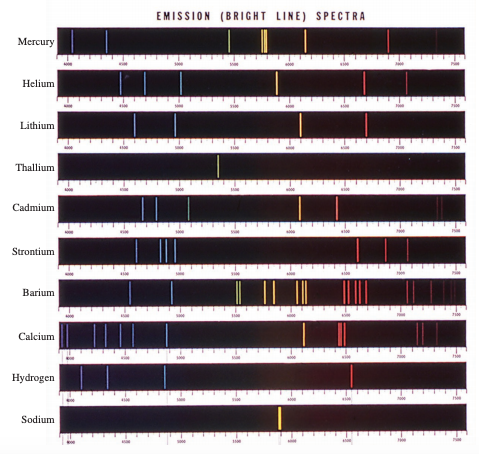# 7.1 - From Classical Physics to Quantum Theory

• We must first grasp the nature of waves to comprehend Planck's quantum theory.

• A wave is a vibrating disturbance via which energy is conveyed.

• The distance between similar spots on successive waves is measured in wavelength.

• The number of waves that travel through a specific place in one second is known as the frequency v (nu).

• The vertical distance between a wave's midline and its peak or trough is called amplitude.

• An electromagnetic wave has an electric field component and a magnetic field component, according to Maxwell's theory.

• The emission and transfer of energy in the form of electromagnetic waves are known as electromagnetic radiation.

• The smallest amount of energy that may be emitted in the form of electromagnetic radiation was given the name quantum by Planck.

# 7.2 - The Photoelectric Effect

• The photoelectric effect is a process in which electrons are ejected from the surface of certain metals when they are exposed to light with a minimum frequency, known as the threshold frequency.

• The lightwave theory is a hypothesis that describes how light travels through space.

• Einstein, on the other hand, made a remarkable assumption.

• He proposed that a light beam is a stream of particles. Photons are the new name for these light particles.

# 7.3 - Bohr’s Theory of the Hydrogen Atom

• Physicists have looked into the properties of emission spectra, or the continuous or line spectra of radiation released by substances.

• Energizing a sample of the material with thermal energy or another source of energy can reveal a substance's emission spectrum.

• Light emission at specific wavelengths is represented by line spectra.• When n = 1, which corresponds to the highest stable energy state, the most negative value is reached.

• This is referred to as the ground state or ground level, and it relates to a system's lowest energy state.

• For n = 2, 3, Each of these levels is referred to as an aroused state or excited level since it is more energetic than the ground state.

# 7.4 - The Dual Nature of the Electron

• An electron bound to the nucleus, according to de Broglie, acts as a standing wave.

• Some spots on the string, known as nodes, do not move at all, implying that the wave amplitude at these points is 0.

# 7.5 - Quantum Mechanics

• Werner Heisenberg developed the Heisenberg uncertainty principle, which states: It is impossible to know the momentum p and the position of a particle with certainty at the same time.

• The wave function of an electron in an atom is known as an atomic orbital.

# 7.6 - Quantum Numbers

• Three quantum numbers are necessary for quantum mechanics to represent the distribution of electrons in hydrogen and other atoms.

• These figures are derived from the Schrödinger equation's mathematical solution for the hydrogen atom.

• The main quantum number, the angular momentum quantum number, and the magnetic quantum number are the three types of quantum numbers.

• These quantum numbers will be used to characterize atomic orbitals as well as to identify electrons that live within them.

# 7.7 - Atomic Orbitals

• The p orbitals begin with the primary quantum number n = 2 as should be obvious.

• There is only a 1s orbital if n = 1. The angular momentum quantum number l can only take the value zero if n = 1.

• When l = 2, there are five different ml values, each of which corresponds to a different d orbital. For a d orbital, the lowest value of n is 3.

# 7.8 - Electron Configuration

• To understand electronic behavior in many-electron atoms, however, we must first understand the atom's electron configuration, or how the electrons are distributed throughout the many atomic orbitals.

• The Pauli exclusion principle is used to determine electron configurations in many-electron atoms.

• No two electrons in an atom may have the same set of four quantum numbers, according to this concept.

• The term "paramagnetic" refers to materials that have net unpaired spins and are attracted to a magnet.

• Diamagnetic materials have no net unpaired spins and are somewhat repelled by magnets.

# 7.9 - The Building-Up Principle

• According to the Aufbau principle, as protons are added one by one to the nucleus to form the elements, electrons are similarly added to the atomic orbitals.

• Except for hydrogen and helium, the electron configurations of all elements are represented by a noble gas core

• which displays in brackets the noble gas element that most nearly precedes the element in question, followed by the symbol for the highest filled subshells in the outermost shells.

• Transition metals either have partially full d subshells or quickly produce cations with partially filled d subshells.